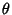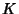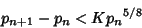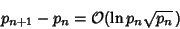## Mills' Constant

N.B. A detailed on-line essay by S. Finch was the starting point for this entry.

Mills (1947) proved the existence of a constantsuch that(1)

is Prime for all, whereis the Floor Function. It is not, however, known ifis Irrational. Mills' proof was based on the following theorem by Hoheisel (1930) and Ingham (1937). Letbe theth Prime, then there exists a constantsuch that(2)

for all. This has more recently been strengthened to(3)

(Mozzochi 1986). If the Riemann Hypothesis is true, then Cramér (1937) showed that(4)

(Finch).

Hardy and Wright (1979) point out that, despite the beauty of such Formulas, they do not have any practical consequences. In fact, unless the exact value ofis known, the Primes themselves must be known in advance to determine. A generalization of Mills' theorem to an arbitrary sequence of Positive Integers is given as an exercise by Ellison and Ellison (1985). Consequently, infinitely many values forother than the numberare possible.

References

Caldwell, C. Mills' Theorem--A Generalization.'' http://www.utm.edu/research/primes/notes/proofs/A3n.html.

Ellison, W. and Ellison, F. Prime Numbers. New York: Wiley, pp. 31-32, 1985.

Finch, S. Favorite Mathematical Constants.'' http://www.mathsoft.com/asolve/constant/mills/mills.html

Hardy, G. H. and Wright, E. M. An Introduction to the Theory of Numbers, 5th ed. Oxford, England: Clarendon Press, 1979.

Mills, W. H. A Prime-Representing Function.'' Bull. Amer. Math. Soc. 53, 604, 1947.

Mozzochi, C. J. On the Difference Between Consecutive Primes.'' J. Number Th. 24, 181-187, 1986.

Ribenboim, P. The Book of Prime Number Records, 2nd ed. New York: Springer-Verlag, pp. 135 and 191-193, 1989.

Ribenboim, P. The Little Book of Big Primes. New York: Springer-Verlag, pp. 109-110, 1991.